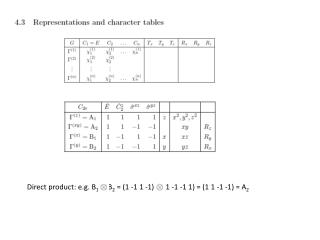DownloadDownload PresentationDirect product: e.g. B 1 B 2 = (1 -1 1 -1) (1 -1 -1 1) = (1 1 -1 -1) = A 2

# Direct product: e.g. B 1 B 2 = (1 -1 1 -1) (1 -1 -1 1) = (1 1 -1 -1) = A 2

Download Presentation## Direct product: e.g. B 1 B 2 = (1 -1 1 -1) (1 -1 -1 1) = (1 1 -1 -1) = A 2

- - - - - - - - - - - - - - - - - - - - - - - - - - - E N D - - - - - - - - - - - - - - - - - - - - - - - - - - -
##### Presentation Transcript

1. Direct product: e.g. B1 B2 = (1 -1 1 -1) (1 -1 -1 1) = (1 1 -1 -1) = A2

2. Two-dimensional representations

3. Only atoms left in place need to be considered. Vectors left unchanged contribute 1 to the character Vectors transformed into their opposite contribute -1 Vectors transformed into other vectors contribute 0

4. Application of the projection formula: construction of normal modes example